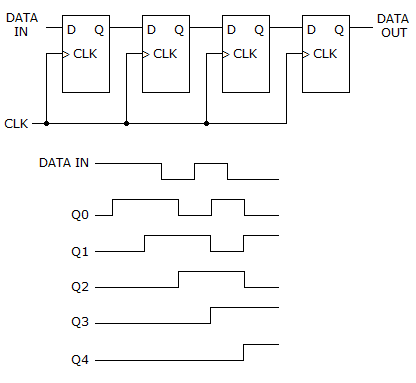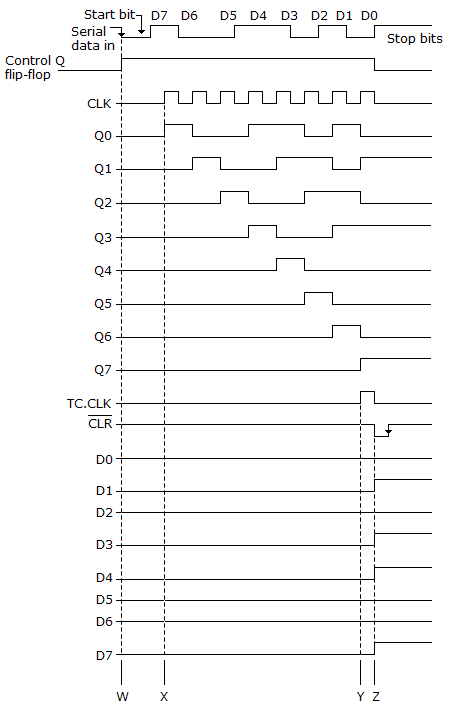# Digital Electronics - Counters

### Exercise :: Counters - General Questions

49.

A counter with a modulus of 16 acts as a ________.

 A. divide-by-8 counter B. divide-by-16 counter C. divide-by-32 counter D. divide-by-64 counter

Explanation:

No answer description available for this question. Let us discuss.

50.

How many data bits can be stored in the register shown below?A. 5 B. 32 C. 31 D. 4

Explanation:

No answer description available for this question. Let us discuss.

51.

What is the difference between a 7490 and a 7493?

 A. 7490 is a MOD-10, 7493 is a MOD-16 B. 7490 is a MOD-16, 7493 is a MOD-10 C. 7490 is a MOD-12, 7493 is a MOD-16 D. 7490 is a MOD-10, 7493 is a MOD-12

Explanation:

No answer description available for this question. Let us discuss.

52.

A ripple counter's speed is limited by the propagation delay of:

 A. each flip-flop B. all flip-flops and gates C. the flip-flops only with gates D. only circuit gates

Explanation:

No answer description available for this question. Let us discuss.

53.

Referring to the given figure, at which point is the serial data transferred to the parallel output?A. W B. X C. Y D. Z

Explanation:

No answer description available for this question. Let us discuss.

54.

A 4-bit counter has a maximum modulus of ________.

 A. 3 B. 6 C. 8 D. 16